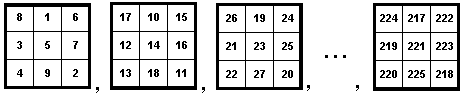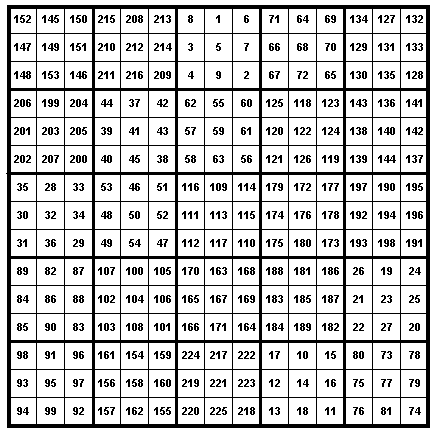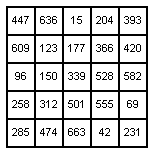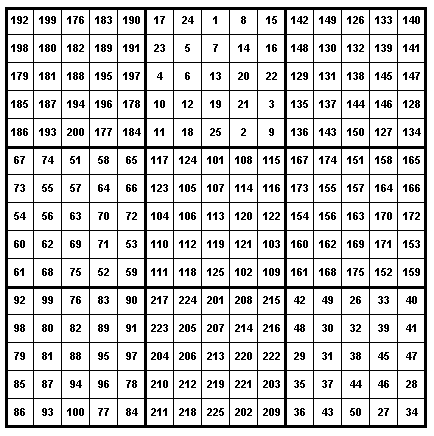Magic Squares within Magic Squares   Some fun magic squares are created using magic squares as the elements of other magic squares. For example we can create a 55 magic square in which the elements are 33 magic squares. The square will be a 1515 magic square using the numbers 1, 2, 3, ... , 225. We create the magic square by first building twenty-five 33 magic squares using the sets {1, 2, 3, ..., 9}, (10, 11, 12, ..., 18}, ..., {215, 218, 219, ..., 225}. That is,We then build a 55 magic square using these twenty-five 33 magic squares as the elements. The 33 magic squares and the 55 magic square are constructed in the usual way for an odd-ordered magic square. The result is the following magic squareIf we replace each of the 33 magic squares by their magic numbers, we get a 55 magic square using the twenty-five numbers in the arithmetic progression {15 + 27*k} for k = 0, 1, 2, ..., 24. That is, the numbers 15, 42, 69, ... ,636, 663 This would give us the magic squareWe can construct magic squares of any order with elements that are also magic squares. We would use the same methods as we do for regular magic squares. An example of a 33 magic square with elements that are 44 magic squares is given below.An example of a 33 magic square with elements that are 55 magic squares is given below.Try some of your own.   | Magic Squares -- Introduction | Magic Squares of Order 3 | 4th Order Magic Squares  | Magic Squares of Order 4n |  Magic Squares of Odd orders | Magic Squares of Even orders--4n+2 | Ben Franklin's Magic Square | Magic Squares which are not Normal |